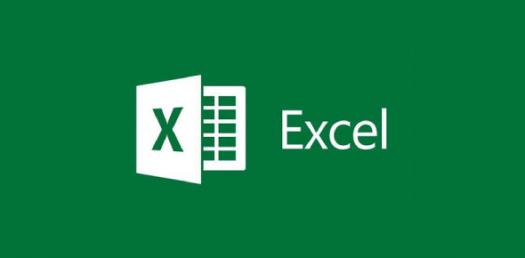# Quiz On Formulas And Functions In Excel! Trivia Questions

21 QuestionsSettings.

Related Topics
• 1.
Active Cells
• A.

• B.

Cell in a spreadsheet were information is placed

• C.

Cells that move alot

• 2.
Autosum
• A.

• B.

Divides selected cells

• C.

Subtracts selected cells

• 3.
Ascending Decimals
• A.

Allows user to multiply decimals

• B.

Allows user to decrease decimals

• C.

Allows user to increase decimals

• 4.
AutoSum
• A.

• B.

• C.

Deletes all cells

• 5.
Cell
• A.

Information is earased in a spreadsheet

• B.

Information is held in a spreadsheet.

• C.

Info is sorted in a spreadsheet

• 6.
• A.

Use this to know the exact location of a specific cell

• B.

Use this to sort all cells

• C.

• 7.
Column
• A.

Horizantal spaces

• B.

Mathematical equation typed into a cell

• C.

These are the vertical spaces

• 8.
Descending Decimals
• A.

User to decrease decimals places of a number typed in a cell. user to decrease decimals places of a number typed in a cell.

• B.

Increase cells

• C.

Allows user to multiply numbers

• 9.
Dollar Symbol
• A.

Symbol

• B.

Changes the contents of a cell to currency

• C.

Number sign

• 10.
Equation
• A.

Displays the cell reference

• B.

Mathematicle typed fourmula

• C.

A mathematical equation typed into a cell.

• 11.
Formula Bar
• A.

Microsoft word tool bar

• B.

Microsoft word number bar

• C.

Microsoft word key board sign

• 12.
Merge and Center
• A.

Move and center align cells

• B.

Function puts the records into a specific order.

• C.

to select a number of cells to create one large cell

• 13.
Microsoft Excel
• A.

A spreadsheet application tool that analyzes data

• B.

A spreadsheet that puts data together

• C.

A spreadsheet that helps you sort all data

• 14.
Name Box
• A.

Diplays title of cells

• B.

Displays the cell reference

• C.

Displays a legend

• 15.
Percent
• A.

Equal amount

• B.

Allows the user to change the value of a cell

• C.

Allows user to change the percent o a cell

• 16.
Row
• A.

Horizantal groups

• B.

Vertical groups

• C.

Diagnal groups

• 17.
Sheettabs
• A.

Lables at the bottom of the screen

• B.

Tabs for tips

• C.

Number card

• 18.
• A.

A document that is used to crunch numbers and formulas.

• B.

A document that is used to create charts.

• C.

A document that is used to create graphs.

• 19.
Sort
• A.

Put in order

• B.

Puts the records into a specific order

• C.

Put things out of order

• 20.
Workbook
• A.

Document that contains 2 worksheets

• B.

Document that contains 4 worksheets

• C.

Document which contains three worksheets

• 21.
Worksheet
• A.

Double sheet that contains an Excel graph

• B.

Single sheet contained in an Excel workbook

• C.

Excel workbook